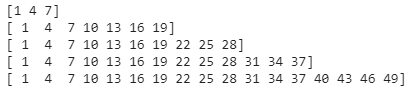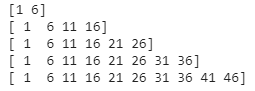# How to Create a Sequence of Linearly Increasing Values with Numpy Arrange?

• Last Updated : 16 Aug, 2022

In this article, we are going to create a sequence of linearly increasing values with Numpy arrange() function.

### Getting Started

By using arrange() function, we can create a sequence of linearly increasing values. This method returns an array with evenly spaced elements as per the interval. The interval mentioned is half opened i.e. [Start, Stop)

Syntax:

`numpy.arrange(start, stop, step)`

Parameters:

• start is the starting value
• stop is the ending value
• step is a linear increment value with in the given range. It is optional. By default, it is 1.

Return Type:

• Return an array of elements

For example:

`numpy.arrange(1,10,3)  # array([1,4,7])`

Here, we have given a range from 1 to 10(start = 1 and stop = 10) but we specified step=3. That means it skips every 3 elements in a range. So In this way, we can increase linearity in data.

Example 1:

Create elements within range with 3-step linearly.

## Python3

 `# importing numpy module``import` `numpy as np` `# create an elements from 1``# to 10 with 3 step linearity``print``(np.arange(``1``, ``10``, ``3``))` `# create an elements from 1``# to 20 with 3 step linearity``print``(np.arange(``1``, ``20``, ``3``))` `# create an elements from 1``# to 30 with 3 step linearity``print``(np.arange(``1``, ``30``, ``3``))` `# create an elements from 1``# to 40 with 3 step linearity``print``(np.arange(``1``, ``40``, ``3``))` `# create an elements from 1``# to 50 with 3 step linearity``print``(np.arange(``1``, ``50``, ``3``))`

Output:Example 2:

Create elements within range with 5-step linearly.

## Python3

 `#importing numpy module``import` `numpy as np` `#create an elements from 1 to``# 10 with 5 step linearity``print``(np.arange(``1``,``10``,``5``))` `#create an elements from 1 to``# 20 with 5 step linearity``print``(np.arange(``1``,``20``,``5``))` `#create an elements from 1 to``# 30 with 5 step linearity``print``(np.arange(``1``,``30``,``5``))` `#create an elements from 1 to``# 40 with 5 step linearity``print``(np.arange(``1``,``40``,``5``))` `#create an elements from 1 to``# 50 with 5 step linearity``print``(np.arange(``1``,``50``,``5``))`

Output:Example 3:

Create elements within the range of 34 to 50 with 5-step linearly.

## Python3

 `#importing numpy module``import` `numpy as np` `#create an elements from 34 to 50 with 4 step linearity``print``(np.arange(``34``,``50``,``5``))`

Output:

`[34 39 44 49]`

My Personal Notes arrow_drop_up